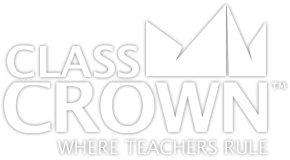# Ratios & Percents Worksheets

## Ratios & Percents Pack 1

Skills included in this pack:
• Determining ratios
• Ratio word problems
• Equivalent ratios
• Proportions
• Proportions word problems
• Similar figures and proportions
• Representing percentages graphically
• Converting between fractions, decimals, and percents
• Converting fractions to percents
• Converting percents to fractions
• Converting between decimals and percents
• Estimating percents using benchmark fractions
• Percent of a number
• Finding percents – numbers of numbers
• Finding a number when a percent of it is known
• Discount and sale prices
• Calculating sales tax
• Percents word problems
• Percents problem solving, mixed
• Ratios and percents, mixed applications
+ See More
Free Sample Pages Available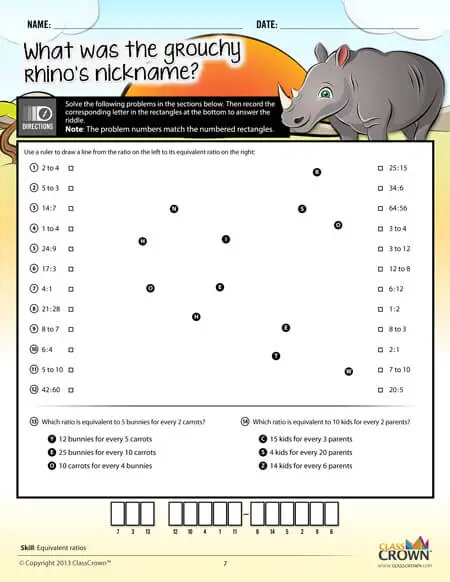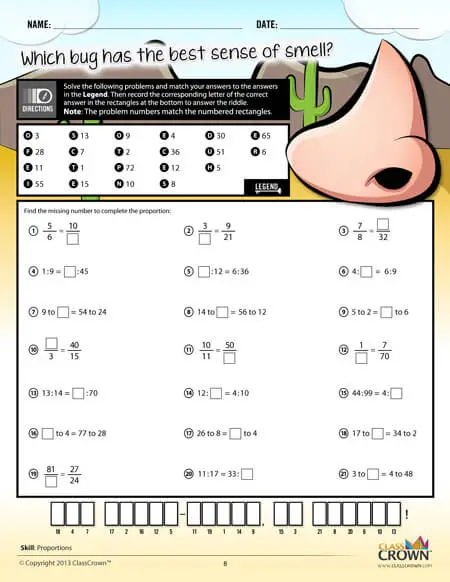Self Checking

Our math worksheets introduce a puzzle aspect to math, giving students immediate feedback as to whether or not they are solving problems correctly. If the answer to the riddle isn't spelled correctly, the student knows which problems he's made an error on.Fun Puzzle AspectImmediate Feedback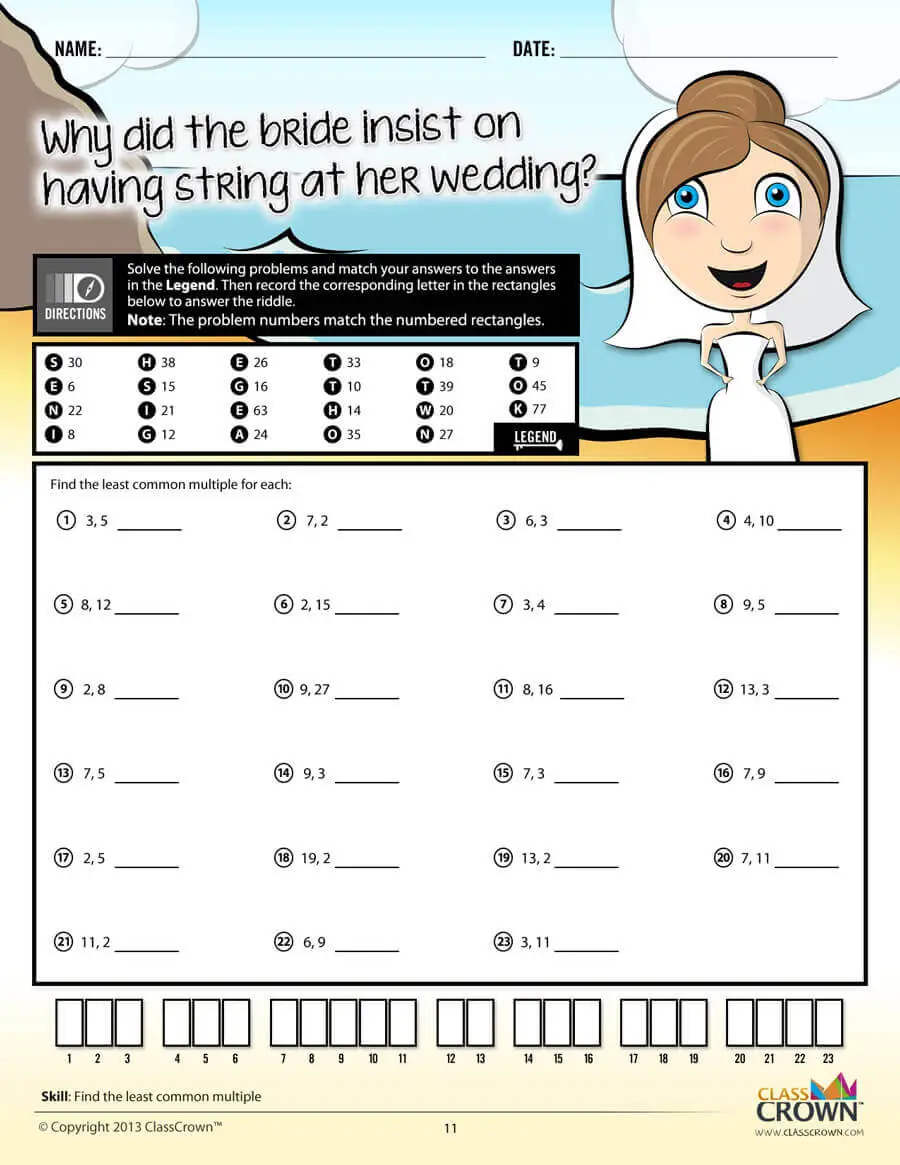Problem Solving Motivation

Each math worksheet contains a riddle that the student solves by completeing all the problems on the worksheet. This keeps kids motivated to complete each problem so that they can find the answer to the riddle.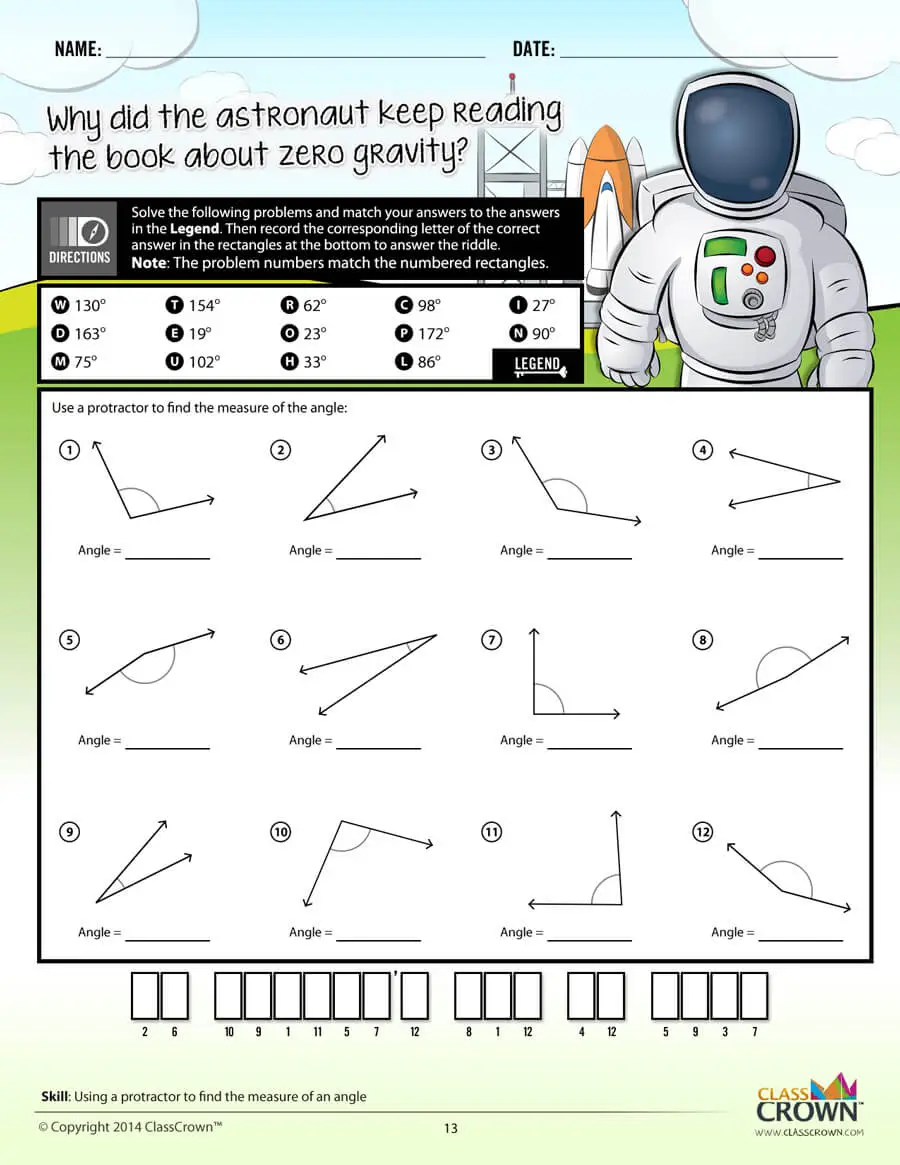Common Core Aligned

All our math worksheet packs are designed with Common Core in mind. That way you don’t have to worry about whether your math ciriculum is aligned or not when you incorpoate ClassCrown Riddle-Me-Worksheets in your lesson plans.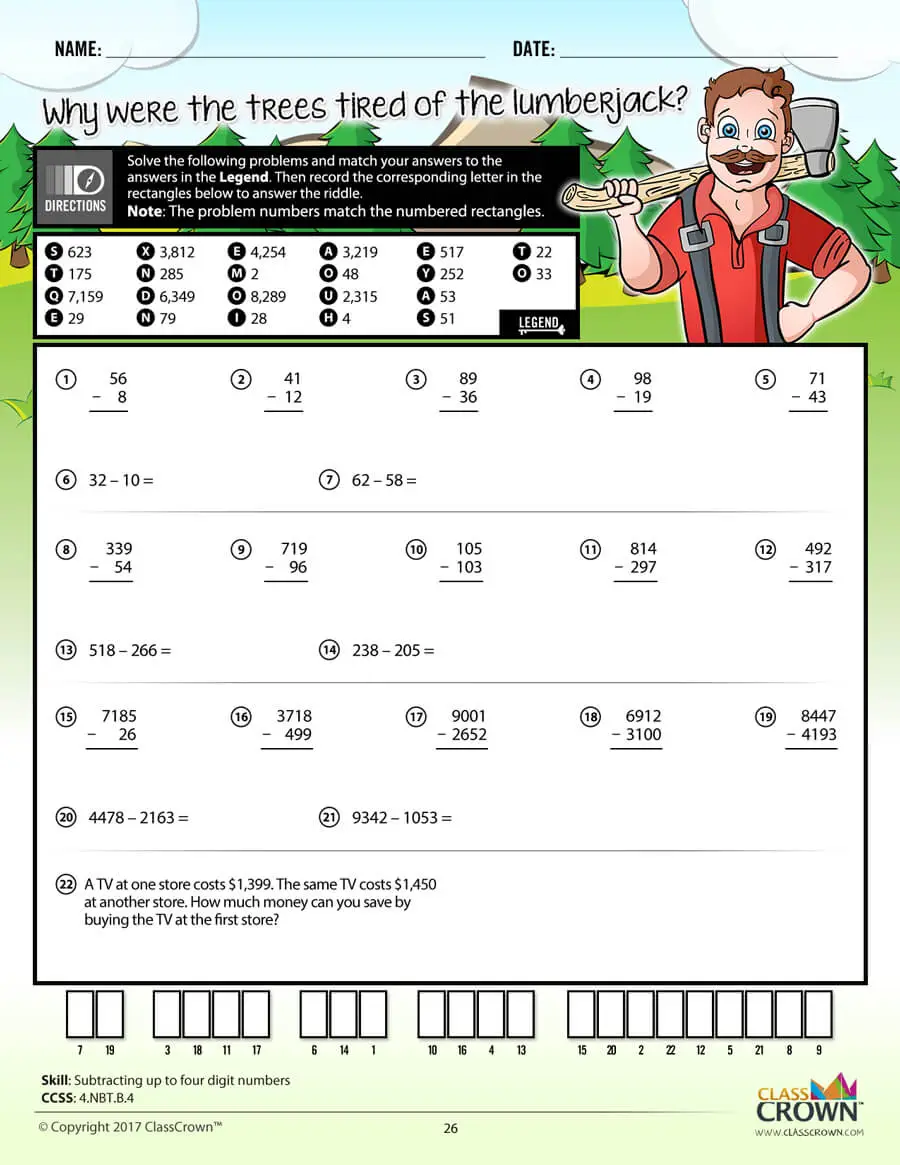High Quality Design

Each page of our math worksheets has been produced in high resolution at 144 dpi and designed in full, vibrant color for maximum quality. They look stunning whether you are printing in color or black and white.High Resolution
(144 dpi)Stunning Color & Clarity